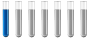## Need help with analytical chemistry Ques

Chemistry and homework help forum.

Organic Chemistry, Analytical Chemistry, Biochemistry, Physical Chemistry, Computational Chemistry, Theoretical Chemistry, High School Chemistry, Colledge Chemistry and University Chemistry Forum.

Share your chemistry ideas, discuss chemical problems, ask for help with scientific chemistry questions, inspire others by your chemistry vision!

Please feel free to start a scientific chemistry discussion here!

Discuss chemistry homework problems with experts!

Ask for help with chemical questions and help others with your chemistry knowledge!

Moderators: expert, ChenBeier, Xen

Saira_Ashraf
NewbiePosts: 1
Joined: Thu Aug 29, 2019 12:12 am

### Need help with analytical chemistry Ques

I am trying to solve this question but in vain if anyone can explain it will be great.

Q: Calculate the solubility of the solutes Th(OH)4 for solution in which the cation concentration is 0.050M.

I found the Ksp which is 1.1*10^-15 and i solved it this way :-

[Th+]+[4OH-]=1.1x10^-15
4[OH]= 4âˆš1.0*10^-15 = 1.8x10^-4
1/4(1.8*10^-4)
=4.5x10^-5 = Final Ans

I have an exam and i am struck with this question pls tell me what is my mistake?
ChenBeier
Distinguished MemberPosts: 742
Joined: Wed Sep 27, 2017 7:25 am
Location: Berlin, Germany
You have mistypings.

Kl = [Th4+] * [(OH-)^4]

Th = x , OH- = 4x

Kl = x* (4x)^4

Kl = 256 * x^5

x =5âˆš (Kl/256)

x = 5âˆš(1.1 * 10^-15/256)

x = 3,36 * 10^-4

OH- = 4*x

OH- = 1,34 * 10^-3 mol/l